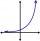# To the cinema

Jane at 8 am got message that all 1093 school pupils will go to the cinema. Within 20 min she said it to the three friends. Each of them again for 20 minutes said to the other three. In this way the message spread further. At what time all the children in school know that they will go to the cinema?

Result

x = 10:20 h#### Solution:We would be pleased if you find an error in the word problem, spelling mistakes, or inaccuracies and send it to us. Thank you!Tips to related online calculators
Do you have a linear equation or system of equations and looking for its solution? Or do you have quadratic equation?

## Next similar math problems:

• Geometric progression 2There is geometric sequence with a1=5.7 and quotient q=-2.5. Calculate a17.
• Six termsFind the first six terms of the sequence a1 = -3, an = 2 * an-1
• Tenth memberCalculate the tenth member of geometric sequence when given: a1=1/2 and q=2
• Terms of GPWhat is the 6th term of the GP 9, 81, 729,. .. ?
• Intercept with axisF(x)=log(x+4)-2, what is the x intercept
• LogarithmDetermine the number whose decimal logarithm is -3.8.
• Five membersWrite first 5 members geometric sequence and determine whether it is increasing or decreasing: a1 = 3 q = -2
• GP - 8 itemsDetermine the first eight members of a geometric progression if a9=512, q=2
• Geometric sequence 4It is given geometric sequence a3 = 7 and a12 = 3. Calculate s23 (= sum of the first 23 members of the sequence).
• Logif ?, what is b?
• Insert into GPBetween numbers 5 and 640 insert as many numbers to form geometric progression so sum of the numbers you entered will be 630. How many numbers you must insert?
• Exponential equationIn the set R solve the equation: ?
• The city 3The city has 22,000 residents. How long it is expected to have 25,000 residents if the average annual population growth is 1.4%?
• SequenceCalculate what member of the sequence specified by ? has value 86.
• Powers 32 to the power of n divided by 4 to the power of -3 equal 4. What is the vaule of n?
• Exponential equationSolve for x: (4^x):0,5=2/64.
• Geometric progressionIn geometric progression, a1 = 7, q = 5. Find the condition for n to sum first n members is: sn≤217.